# Children 9

There are 11 children in a room.

6 of the children are girls.

What fraction of the children are girls?

x =  0.5455

### Step-by-step explanation: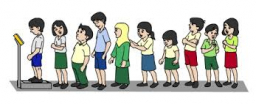Did you find an error or inaccuracy? Feel free to write us. Thank you!Tips to related online calculators
Need help to calculate sum, simplify or multiply fractions? Try our fraction calculator.

## Related math problems and questions:

• There 12There are 42 students in the class and 2/3 of them are girls. How may girls are there in the class?
• A class IV.AIn a class there are 15 girls and 30 boys.  What fraction of the class represents the boys?
• The ratioThe ratio of girls to boys in Mrs. White's class is 3:2.  If there are 12 boys, how many girls are in the class room?
• Babies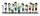There are 2 adults 2 children and 4 babies on a bus. What fraction of the people are babies?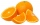If there are 7 apples and 5 oranges in the basket then what fraction of oranges are there in the fruit basket?
• Camp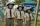In the camp are children. 1/2 went on a trip, 1/4 went to bathe, and 38 children remained in the room. How many children are in the camp?
• StudentsThere are 2/6 girls out of 1050 students at the school. Of these, 4/5 have another sibling. How many girls are in school? How many boys are in school? How many girls do not have a sibling?
• There 13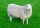There are 7 cows 3 sheeps and 4 chickens on a field . What fraction of the animals are sheep?
• Group of childrenThere is a group of children. There is a boy named Adam in each of the three children subgroup and a girl named Beata in each quartet (four-member subgroup). How many children can be in such a group and what are their names in that case?
• In the cafeteriaThere are 18 students in Jacob’s homeroom. Six students bring their lunch to school. The rest eat lunch in the cafeteria. In simplest form, what fraction of students eat lunch in the cafeteria?
• FractionFind for what x fraction (-4x -6)/(x) equals:
• Pupils 7There are 40 pupils in a certain class. 3/5 of the class are boys. How many are girls?
• Dining roomThe dining room has 11 tables (six and eight seats). In total there are 78 seats in the dining room. How many are six-and eight-seat tables?
• Boys and girlsThere are 28 girls in the hall. 5/7 of all children are boys. How many children and how many boys are there?
• ChildrenThe group has 42 children. There are 4 more boys than girls. How many boys and girls are in the group?
• GroupsIn the 6th class there are 60 girls and 72 boys. We want to divide them into groups so that the number of girls and boys is the same. How many groups can you create? How many girls will be in the group?
• What fraction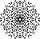What fraction of number 1 to 30 is prime?